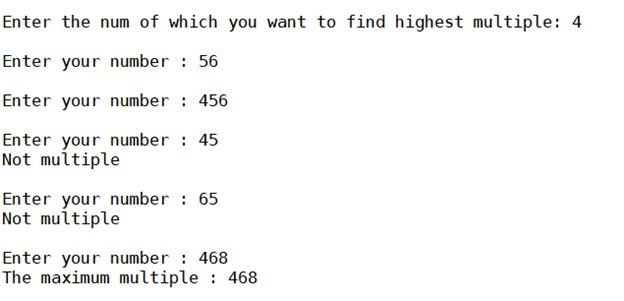# Python program to find the maximum multiple from given N numbers

Here, we are going to find the maximum multiple from the given N numbers using python program.
Submitted by Anuj Singh, on September 14, 2019

Here, we will be framing code for finding the maximum multiple of a number x from a given set of a number (set of 5 numbers in this program).

There are many ways of doing this but this time, we have to thought of most computationally efficient algorithm to do so.

Following is the code for such problem,

```n = 0

num = 0

maxnum = 0

x = int(input("Enter the num of which you want to find highest multiple: "))

while n<5:
num = int(input("Enter your number : "))
if num%x == 0:
if num > maxnum:
maxnum = num
else:
print("Not multiple")

n += 1

print("The maximum multiple :",maxnum)
```

OutputPreparation

What's New

Top Interview Coding Problems/Challenges!